Subsections

# MA 1024 Lab 4: Partial derivatives, directional derivatives, and the gradient

## Purpose

The purpose of this lab is to acquaint you with using Maple to compute partial derivatives, directional derivatives, and the gradient.

## Background

For a function f(x) of a single real variable, the derivative f'(x) gives information on whether the graph of f is increasing or decreasing. Finding where the derivative is zero was important in finding extreme values. For a function of two (or more) variables, the situation is more complicated.

### Partial derivatives

A differentiable function, F(x,y), of two variables has two partial derivatives: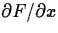and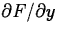. As you have learned in class, computing partial derivatives is very much like computing regular derivatives. The main difference is that when you are computing, you must treat the variable y as if it was a constant and vice-versa when computing.

The Maple commands for computing partial derivatives are D and diff. The Getting Started worksheet has examples of how to use these commands to compute partial derivatives.

### Directional derivatives

The partial derivativesandof F can be thought of as the rate of change of F in the direction parallel to the x and y axes, respectively. The directional derivative, where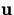is a unit vector, is the rate of change of F in the direction. There are several different ways that the directional derivative can be computed. The method most often used for hand calculation relies on the gradient, which will be described below. It is also possible to simply use the definition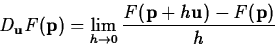to compute the directional derivative. However, the following computation, based on the definition, is often simpler to use.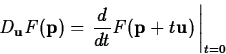One way to think about this that can be helpful in understanding directional derivatives is to realize thatis a straight line in the x,y plane. The plane perpendicular to the x,y plane that contains this straight line intersects the surface z = F(x,y) in a curve whose z coordinate is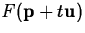. The derivative ofat t=0 is the rate of change of F at the point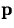moving in the direction.

Maple doesn't have a simple command for computing directional derivatives. There is a command in the tensor package that can be used, but it is a little confusing unless you know something about tensors. Fortunately, the method described above and the method using the gradient described below are both easy to implement in Maple. Examples are given in the Getting Started worksheet.

### The Gradient

The gradient of F, written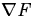, is most easily computed as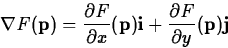As described in the text, the gradient has several important properties, including the following.
• The gradient can be used to compute the directional derivative as follows.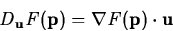• The gradient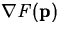points in the direction of maximum increase of the value of F at.
• The gradientis perpendicular to the level curve of F that passes through the point.
• The gradient can be easily generalized to apply to functions of three or more variables.

Maple has a fairly simple command grad in the linalg package (which we used for curve computations). Examples of computing gradients, using the gradient to compute directional derivatives, and plotting the gradient field are all in the Getting Started worksheet.

## Exercises

1.
Compute the four second order partial derivatives of the following functions using the indicated Maple command.
(a), using diff.
(b)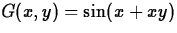, using D.
2.
Consider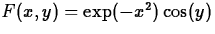. Compute the directional derivative of F at the pointin the direction of the vector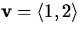using each of the two methods demonstrated in the Getting Started worksheet. Maple will probably not simplify the answer unless you tell it to. Use either the value command or the simplify command to show that the directional derivative is zero. Explain this answer geometrically in terms of the vectorand the contour of F that passes through the point.

3.
Consider the simple function F(x,y) = 5-x2-2y2. It is easy to discover by plotting this function that it has a maximum value of 5 at the origin. Demonstrate, with plots of the gradient field and an explanation, how the gradient field can also be used to locate (approximately) where the maximum occurs.

4.
Compute the gradient of the function

H(x,y) = x3-3x-y3+3y

and plot the gradient field over the domain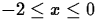and. Use your plot to find the approximate location of the maximum value of this function over this domain.

5.
In a previous lab, you used contour plots to get information about the behavior of the graph of a function of two variables. Use the technique demonstrated in the Getting Started worksheet to plot the gradient field and a contour plot on the same graph for the function H(x,y) from the previous exercise. Use the same domain ofand. Which plot (contour plot or gradient field plot) do you think gives you more information about the behavior of the function? Explain your answer.Next: About this document ... Up: No Title Previous: No Title

Jane E Bouchard
4/19/2000